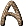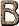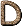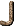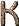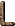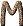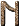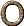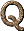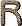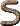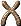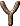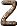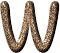W3 A dense cloud of gas about 3 kpc distant in the Perseus arm. [H76] W44 A radio source. It is a supernova remnant about 3 kpc distant and less than 0°.5 from the galactic plane. [H76] W49 A radio source (a giant H II region) about 14 kpc distant. It is the most powerful thermal radio source known in our Galaxy. [H76] W51 A radio source, a supernova remnant. PSR 1919+14 lies within its radio contours. [H76] W and Z Particles Particles that transmit the unified electromagnetic and weak nuclear forces. These particles were predicted by the Weinberg-Salam theory of the 1960s and later discovered in the 1980s. (see Electroweak Theory; Weinberg-Salam Theory.) [LB90] W Boson see Intermediate Vector Boson.[H76] Warp The deviation from flatness in the outer Galactic disk. Some parts of the outer disk lie above the Galactic plane; others lie below it. [C95] Watt The SI unit of power. 1 W = 107 ergs s-1. [H76] Wave A propagating pattern of disturbance. One example is a sound wave, in which a pattern of alternating high and low pressures propagates through air. Another example is an electromagnetic wave, in which a pattern of electric and magnetic fields propagates through empty space. [G97] Wave Function (a) The mathematical object in quantum theory which determines probabilities of different results of experiments. It is a complex quantity, so it has an amplitude (whose square gives the probability) and a phase-angle. The phase-angle has no direct physical interpretation, but is important in interference effects, where two wave-functions are added together. [D89] (b) The mathematical description of a physical system according to the laws of quantum mechanics. The wave function tells what possible states the physical system could be in and what is the probability of being in any particular state at any given moment. [LB90] (c) A mathematical function that describes the wave-mechanical state of a system (atomic or nuclear). In a one-electron atom, it yields the likelihood that the electron will be found in the neighborhood of that point (per unit volume). This interpretation can be generalized to more complicated systems. [H76] Wavefront A continuous surface associated with a wave radiation, in which all the vibrations concerned are in phase. A parallel beam has plane wavefronts; the output of a point source has spherical wavefronts. [DC99] Wavefront Error The departure of the true wavefront in an electromagnetic wave propagating through an optical system from the ideal spherical wave at that point. [McL97] Wavelength (a) The distance between adjacent peaks in a wave-train is the wavelength. [D89] (b) A mathematical functiondescribing the behavior of a particle according to quantum mechanics. The wave function satisfies Schrodinger's wave equation. Furthermore, the probability of finding the particle at a particular point in space is given by the absolute square of the wave function. [CD99] (c) The distance between two successive peaks (often called crests) of the propagating disturbance of a wave. [G97] (d) In electromagnetic radiation, the distance between successive crests of the "waves" radiated. The number of such crests passing a fixed point in 1 second is known as the frequency (a crest per second = 1 Hertz); a short wavelength thus implies high frequency. Of light, the unit of wavelength is the angstrom (Å), measured as 10-10 meters. Visible light is of between 4,000 and 7,500 Å; different wavelengths are perceived by the eye as the different colors of the spectrum. Radio wavelengths, however, may measure from about 50 cm to about 10,000 m. [A84] Wave Mechanics A quantum-mechanical theory introduced by Schrödinger in 1926 which ascribes wave characteristics to the fundamental entities of atomic structure, and formulates the appropriate wave equation (Schrödinger's equation). [H76] Wave Noise Noise in the current of a detector, caused by fluctuations in the electromagnetic radiation falling on the detector (cf. shot noise). [H76] Wave Number (a) Symbol:The reciprocal of the wavelength of a wave. It is the number of wave cycles in unit distance, and is often used in spectroscopy. The unit is the meter-1 (m-1). The circular wave number (symbol: k) is given by: k = 2(b) The reciprocal of the wavelength; i.e., the number of waves per unit distance in the direction of propagation. [H76] (c)The name adopted by the British Association in 1872 to replace reciprocal wavelength in spectroscopy. In its original form the wavenumber represented the number of wavelengths per millimetre but today it is the number of wavelengths per centimetre. The name was proposed by G. J. Stoney and J. E. Reynolds in 1871. [JM92] Wave-Particle Duality (a) Quantum realization that particles of matter and energy also exhibit many of the characteristics of waves. [F88] (b) Basic feature of quantum mechanics that objects manifest both wavelike and particle-like properties. [G99] Wave Zone The field of a pulsar beyond the velocity-of-light radius. (also called Far Fie ) [H76] Waves Propagation of energy by means of coherent vibration. [F88] Weak-Equivalence Principle A principle derived from the equality of the inertial and gravitational mass, which states that if we observe two bodies experiencing equal accelerations, we cannot, by observing the motion, tell whether they are being subjected to a uniform acceleration by some external mechanism or whether they happen to be in a uniform gravitational field. [H76] Weak-Field Condition (gravitational)<< c2, whereis the Newtonian gravitational potential. [H76] Weak (Nuclear) Force One of the four fundamental forces, best known for mediating radioactive decay. [G99] Weak G-band Stars A G-type giant (G5 to K5) with a very weak or absent G band of CH and weak CN bands. These stars are C-deficient. [JJ95] Weak Gauge Boson Smallest bundle of the weak force field; messenger particle of the weak force; called W or Z boson. [G99] Weak Gauge Symmetry Gauge symmetry underlying the weak force. [G99] Weak Interaction (a) One of the fundamental forces of nature. Its most famous manifestation is in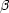-decay; it is also involved in some radioactive decays of nuclei, and neutrino interactions. [D89] (b) A short-range nuclear force responsible for radioactivity and for the decay of certain unstable nuclei, e.g., e- + p <=> n + ve, which is so called because it occurs at a rate slower than that of the strong interaction by a factor of about 10-13 (see Interactions). [H76] (c) Interactions with a typical range of about 1/100 the size of an atomic nucleus which are responsible for the beta decay of some kinds of an atomic nuclei. Neutrinos are subject to only the weak interactions and the much weaker force of gravity. [G97] Weak-Line Stars Late type objects in which the lines of all metals are weakened when compared with normal stars of the same temperature. Also called metal-weak stars. [JJ95] Weak Nuclear Force One of the four fundamental forces of nature. Controls the interaction of neutrinos. [c97] Weakly Coupled Theory whose string coupling coyistant is less than 1. [G99] Weber (Wb) The derived SI unit of magnetic flux. 1 weber = 108 maxwells. [H76] Weber Bar gravitational-wave detector pioneered by Joseph Weber, consisting of a solid aluminum cylinder, suspended and isolated from surrounding vibrations. The cylinder can weigh several tons. [D89] Week An arbitrary period of days, usually seven days; approximately equal to the number of days counted between the four phases of the Moon. (see Lunar Phases) [S92] Weinberg Angle (w) parameter in the electroweak theory. Relates properties, such as mass, of the W and Z bosons and their interactions. [D89] Weinberg-Salam Model (a) An alternative name for the Electroweak Theory. [D89] (b) A theory in physics, developed by Steven Weinberg, Abdus Salam, and Sheldon Glashow, that unifies two fundamental forces of nature, the electromagnetic force and the weak nuclear force. This is the same as the Electroweak Theory. [LB90] Werner Lines Spectral lines of molecular hydrogen in the ultraviolet, in the same general region as the Lyman lines. [H76] Wesselink Analysis A method of determining the radius of a variable star. (also known as the Baade-Wesselink Method [H76] Wheatstone Bridge A device that measures the resistance of an electrical circuit to the flow of electricity. [LB90] Whirlpool Galaxy A spiral galaxy (M51, NGC 5194) of type Sc in Canes Venatici. [H76] Whistlers Radio waves generated by a flash of lightning, which travel along Earth's magnetic field out beyond the ionosphere and back to Earth. They arrive back with a descending pitch because the high-frequency end of the wave train arrives first (see Dispersion). [H76] White Dwarf (a) Compact star with mass less than about 1.4 solar masses, typical radius of 1000 km; supported against gravity by quantum-mechanical degeneracy pressure of electrons. [D89] (b) A small, faint, dense, dying star that has used up its nuclear fuel and is slowly fading from view. A typical white dwarf has 60 percent of the Sun's mass but is little larger than the Earth. White dwarfs are common, accounting for 10 percent of all stars in the Galaxy; the nearest is Sirius B, just 8.6 light-years away. But no white dwarf is visible to the naked eye. [C95] (c) A star of high surface temperature, low luminosity, and high density (105-108 g cm-3), with roughly the mass of the Sun and the radius of the Earth, that has exhausted most or all of its nuclear fuel, believed to be a star near its final stage of evolution. When the Sun becomes a white dwarf, its radius will be about 0.01 of its present radius. DA white dwarfs are hydrogen-rich; DB white dwarfs are helium-rich; DC are carbon rich; DF are calcium-rich; DP are magnetic stars. White dwarfs have relatively low rotational velocities. [H76] White Giant A giant star of spectral type A. Some RR Lyrae stars are white giants. (rarely used term) [C95] White Hole The time-reversal of a black hole. A white hole is a singularity from which matter emerges unpredictably, but into which matter cannot enter. The initial singularity of the standard big bang theory is an example of a white hole. It can be shown that the creation of a new universe from a false vacuum bubble in the context of classical General Relativity would require a white hole singularity, which means essentially that it cannot be done, even in principle. However, a false vacuum bubble could conceivably grow to become a new universe through a process of quantum tunneling. [G97] White Light Visible radiation that gives a sensation of whiteness. The effect is very subjective and depends very much upon conditions and contrast. Light from a lamp with filament temperature about 2000K may be regarded as white, but in contrast to a filament at 3000K it appears yellow. Sunlight normally appears white, but a small beam of sunlight entering a room lit mainly by filament lamps can appear blue-green. Yet the Sun is sometimes classified as a yellow star, in contrast to some stars with radiating surfaces at higher temperature. In all the above cases the effect is caused by a continuum over the whole visible spectrum. The sensation of whiteness can also be produced by suitable combinations of parts of the spectrum; for example, two complementary colors or three primary colors. [DC99] White Noise Completely random and uncorrelated noise, with equal power at all frequencies. [Silk90] White Supergiant A supergiant star with a spectral type of A. White supergiants are rare; the nearest is Deneb, which lies 1500 light-years away. (rarely used term) [C95] Widmanstätten Pattern A geometric pattern found in some iron meteorites, consisting of groups of parallel lamellae crossing each other at various angles. [H76] Wien's Displacement Law For black body radiation the rate of energy radiation per unit area per unit wavelength range at constant kelvin temperature T1 can be plotted against wavelength. It is found that there is a peak at wavelength1. For temperature T2 the peak comes at2, such that1 T1 =2 T2 = constant This rule was deduced by Wien (1893) by thermodynamics, but he could not evaluate the constant nor explain the shape of the graph. The problem was solved by Planck. (see Planck's Radiation Law) [DC99] Wien's Law The wavelength at which a blackbody emits the greatest amount of radiation is inversely proportional to its absolute temperature. [H76] Wilson-Bappu Effect A linear relation between the width of the K2 emission core in the resonance line of Ca II at 3933 Å, detectable for late-type stars, and the absolute magnitudes of the stars. [H76] WIMPWeakly Interacting Massive Particle (a) Some astronomers believe that these exotic subatomic particles make up most of the mass of the Universe. [C95] (b) A weakly interacting massive particle. A generic term for a class of hypothetical particle which may form the missing mass. A form of non-baryonic cold dark matter. [c97] Winding Energy The energy embodied by a string wound around a circular dimension of space. [G99] Winding Mode A string configuration that wraps around a circular spatial dimension. [G99] Winding Number The number of times a string is wound around a circular spatial dimension. [G99] Window A term used to describe the spectral range within which the Earth's atmosphere is transparent to radiation (see Optical Window; Radio Window). Earth's atmosphere is completely opaque to X-rays; ultraviolet radiation is absorbed by electronic transitions in the ozone layer, but it is possible to get above some of it in balloons and rockets. Infrared radiation is absorbed by water vapor, so a high mountain or desert will let some radiation through (the vibrations of molecules cause absorption in the near-infrared, and the rotation of molecules causes absorption in the far-infrared and short radio). Wavelengths beyond the radio window are absorbed by the ionosphere. [H76] WINO The supersymmetric partner of the W boson [K2000] WKB method (Wentzel-Kramers-Brillouin) A method for obtaining an approximate solution to Schrödinger's equation. [H76] WLRG Weak-Lined Radio Galaxy Wolf Diagram Logarithmic plot of N (number of stars or galaxies counted at successive magnitude limits) versus apparent magnitude. [H76] Wolf-Lundmark-Melotte System A dwarf E5 elliptical galaxy, sometimes considered a member of the Local Group. (also known as WLM) [H76] Wolf Number (R) (Also called the Relative Number) A quantity which gives the number of sunspots, and the number of groups of sunspots, at a given time. R = k (10g + f) where k is a constant depending on observing conditions, g is the number of sunspot groups, and f is the number of individual spots visible on the Sun at a given time. [H76] Wolf-Rayet (WR) Star One of a class of very luminous, very hot (as high as 50,000 K) stars whose spectra have broad emission lines (mainly He I and He II), which are presumed to originate from material ejected from the star at very high (~ 2000 km s-1 ) velocities. Some W-R spectra show emission lines due to carbon (WC stars); others show emission lines due to nitrogen (WN stars). (Hiltner and Schild classification: WN-A, narrow lines; WN-B, broad lines.) [H76] Wollaston Prism A prism used to obtain plane-polarized light. [H76] Work Function (W): The amount of energy needed to release an electron from the attraction of positive ions in a metal. It is different for different metals. [H76] World Line (a) In space-time, the history of a particle is represented by a world-line. The position of the particle in space at any particular time t is found by slicing space-time at time t and seeing where the slice cuts the world-line. [D89] (b) The trajectory of a body moving through spacetime. [c97] (c) In relativity, the path traced out in four-dimensional space-time by a given object or particle. [F88] (d) The graph in spacetime coordinates which represents any continuous sequence of events relating to a given particle. In general relativity, all material particles have timelike world lines, photons have null world lines, and tachyons have spacelike world lines. [H76] World Model A mathematical model of the Universe. [H76] World Point see Event. [H76] World Surface/World Sheet The surface traced in space-time by an extended object such as a string. [P88] Wormhole(a) A bridge to another universe created by a black hole. [LB90] (b) An intriguing solution to the equations of general relativity which describes a neck that can connect two completely separate universes. Wormholes arise in the discussion of the creation of a universe in the laboratory, because the new universe disappears through a wormhole and completely detaches itself from the parent universe. [G97] (c) A tube-like region of space connecting one region of the Universe to another. [G99] W particle (a) Very massive charged (+ or -) particle that conveys part of the weak force between leptons and hadrons. [D89] (b) Massive bosons thought to have been abundant in the early Universe, when the unified electroweak force was manifest. [F88] W Velocity A star's velocity perpendicular to the Galactic plane. If a star is moving up, its W velocity is positive; if a star is moving down, its W velocity is negative; and if a star does neither, its W velocity is zero. The Sun has a W velocity of +7 kilometers per second, so it is moving up at 7 kilometers per second. In general, the greater a star's W velocity when it crosses the Galactic plane, the farther above and below the plane the star will travel. [C95] W Virginis Star Also known as a population II Cepheid, a W Virginis star is a bright yellow star that pulsates like a Cepheid but is older and fainter. W Virginis stars appear in globular clusters. [C95] WR or Wolf-Rayet stars Hot stars characterized by wide emission lines of highly ionized elements, standing out distinctly from the continuous spectrum. There exist three varieties: WN exhibit emission lines of NIII, NIV or NV WC exhibit emission lines of CII, CIII or CIV WO exhibit emissions of OIV, OV or OVI [JJ95] [WR] stars Wolf-Rayet stars that are the central objects of a planetary nebulae. [JJ95]